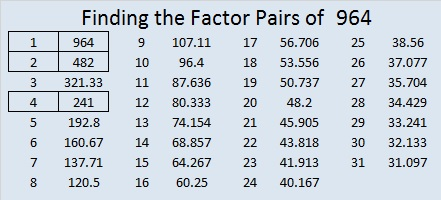1030 Cupid’s Arrow

Love can often be like a puzzle. When cupid’s arrow hits its mark, at first everything might seem to fall into place, but before long, love starts getting complicated and has to be figured out.

That’s the way this Cupid’s Arrow puzzle is, too. It’s easy to find the logic to start it, but then the logic will be more difficult to see. May you be able to figure out this puzzle as well as the important relationships in your life!Print the puzzles or type the solution in this excel file: 12 factors 1028-1034

What can I tell you about the number 1030?

It’s the sum of two consecutive prime numbers:
509 + 521 = 1030

It’s the hypotenuse of a Pythagorean triple:
618-824-1030 which is (3-4-5) times 206

It’s palindrome 1102011 in BASE 3 because 3⁶ + 3⁵ + 2(3³) + 3¹ + 3⁰ = 1030

• 1030 is a composite number.
• Prime factorization: 1030 = 2 × 5 × 103
• The exponents in the prime factorization are 1, 1, and 1. Adding one to each and multiplying we get (1 + 1)(1 + 1)(1 + 1) = 2 × 2 × 2 = 8. Therefore 1030 has exactly 8 factors.
• Factors of 1030: 1, 2, 5, 10, 103, 206, 515, 1030
• Factor pairs: 1030 = 1 × 1030, 2 × 515, 5 × 206, or 10 × 103
• 1030 has no square factors that allow its square root to be simplified. √1030 ≈ 32.09361964 Bow and Arrow

You can google lots of images of bows and arrows related to Thanksgiving. Perhaps that is because Indians from the Wampanoag Nation joined the Pilgrims in what we call the first Thanksgiving. I’m sure you know the story very well told from the white man’s point of view. It will be well worth your time to read The REAL Story of Thanksgiving as well. Everyone’s History Matters is also an excellent read.Print the puzzles or type the solution in this excel file: 12 factors 959-967

Now I’ll tell you a little about the number 964:

964 is the sum of the fourteen prime numbers from 41 to 101.

It is also the sum of four consecutive prime numbers:
233 + 239 + 241 + 251 = 964

30² + 8²  = 964 making it the hypotenuse of a Pythagorean triple:
480-836-964 which is 4 times (120-209-241)

964 is a palindrome in 2 bases and a repdigit in another.
1022201 in BASE 3
7A7 in BASE 11 (A is 10 in base 10)
444 in BASE 15

• 964 is a composite number.
• Prime factorization: 964 = 2 × 2 × 241, which can be written 964 = 2² × 241
• The exponents in the prime factorization are 2 and 1. Adding one to each and multiplying we get (2 + 1)(1 + 1) = 3 × 2  = 6. Therefore 964 has exactly 6 factors.
• Factors of 964: 1, 2, 4, 241, 482, 964
• Factor pairs: 964 = 1 × 964, 2 × 482, or 4 × 241
• Taking the factor pair with the largest square number factor, we get √964 = (√4)(√241) = 2√241 ≈ 31.048349963 Arrow

The numbers 1 to 12 fit someplace in the first column as well as in the top row.  Can you figure out where those places are so that this puzzle can become a multiplication table?Print the puzzles or type the solution in this excel file: 12 factors 959-967

Now I’d like to mention a few things about the number 963:

It is the sum of the 24 prime numbers from 2 to 89. Pretty cool!

I like the way 963 looks in base 10 as well as in a few other bases:
33003 in BASE 4 because 3(4⁴) + 3(4³) + 3(1) = 3 × 321 = 963
3C3 in BASE 16 (C is 12 in base 10) because 3(16²) + 12(16) + 3(1) = 963
1B1 in BASE 26 (B is 11 in base 10) because 1(26²) + 11(26) + 1(1) = 963
123 in BASE 30 because 1(30²) + 2(30¹) + 3(30⁰) = 963

• 963 is a composite number.
• Prime factorization: 963 = 3 × 3 × 107, which can be written 963 = 3² × 107
• The exponents in the prime factorization are 2 and 1. Adding one to each and multiplying we get (2 + 1)(1 + 1) = 3 × 2  = 6. Therefore 963 has exactly 6 factors.
• Factors of 963: 1, 3, 9, 107, 321, 963
• Factor pairs: 963 = 1 × 963, 3 × 321, or 9 × 107
• Taking the factor pair with the largest square number factor, we get √963 = (√9)(√107) = 3√107 ≈ 31.03224